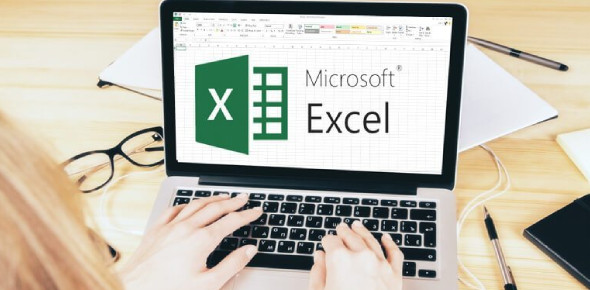# How Well Do You Know MS Excel?

21 Questions | Attempts: 404SettingsMicrosoft Excel plays a key role in many sectors. It is used for spreadsheets in a lot of business activities, and the organization of personal data. Ever wondered how much you know about MS Excel? Take the online quiz and find out.

• 1.
_______, ________ and _______ are what you see when you open Excel
• A.

Columns, rows, and charts

• B.

Columns, rows, and formulas

• C.

Columns, rows, and cells

• D.

Non of the above

• 2.
When you start Excel, you open a file that's called a workbook. Each new workbook comes with_____________ worksheet(s), like pages in a document. You enter data into the worksheets. (Worksheets are sometimes called spreadsheets.)
• A.

1

• B.

2

• C.

3

• D.

4

• 3.
___________ are where you get down to business and enter data in a worksheet.
• A.

Cells

• B.

Fields

• C.

Tables

• D.

None of the above

• 4.
You can use Excel to create budgets, to work with taxes, or to record student grades
• A.

True

• B.

False

• C.

Option 3

• D.

Option 4

• 5.
A formula result is in cell C6. You wonder how you got the result. To see the formula, you:
• A.

Click in cell C6, and then press CTRL+SHIFT.

• B.

Click in cell C6, and then press CTRL+SHIFT.

• C.

Click in cell C6.

• D.

Non of the above

• 6.
I am result of adding two or more numbers. Who am i?
• 7.
To divide 853 by 16 in a formula in Excel, you would use what math operator?
• 8.
I plays na important role in performing any calculation, for any formula or as logical operator in excel. Who am i.. (used symbol not word)
• 9.
The cell in the worksheet in which you can type data is called the stem cells.
• A.

True

• B.

False

• 10.
A1=10 B2=20 C3=30  BASED ON THE GIVEN DATA. USING THE MS EXCEL A1+B2=30? THE EXPRESSION IS TRUE?
• A.

True

• B.

False

• 11.
RESULT ARE THE NUMBERS ENTERED IN A CELL?
• A.

True

• B.

False

• 12.
Product is the sum of two numbers
• A.

True

• B.

False

• 13.
Quotient is the product of the sum of two numbers
• A.

True

• B.

False

• 14.
Numbers and Letters are called Data
• A.

True

• B.

False

• 15.
=A1-B2= Difference
• A.

True

• B.

False

• 16.
A1:20 defines as the range of the value of A1 to A20
• A.

True

• B.

False

• 17.
In MS Excel +,-,*,/ are called Mathematical operation while Addition, Subtraction, Multiplication, Division are called Symbols
• A.

True

• B.

False

• 18.
(Asterisk) is used as the 'Multiplication operator' in MS Excel
• A.

True

• B.

False

• 19.
In MS Excel, A Data  is an equation that calculates a new value from values currently in a worksheet
• A.

True

• B.

False

• 20.
Which one do you like?
• A.

Option 1

• B.

Option 2

• C.

Option 3

• D.

Option 4

## Related TopicsBack to top
×

Wait!
Here's an interesting quiz for you.